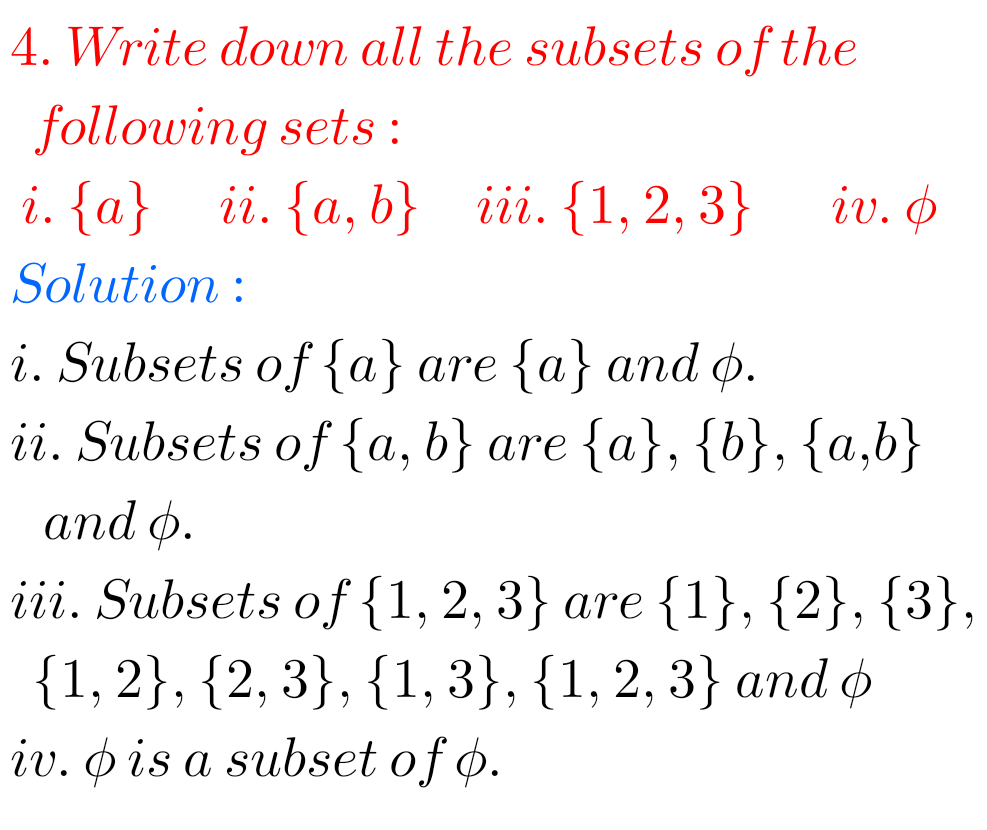# solutions for class 11 th mathematics## NCERT Maths solutions class 11 Chapter 1 Sets Exercise 1.3

Sets Exercise 1.3 solutions Class 11 NCERT maths NCERT Mathematics class 11 Chapter 1 Sets Exercise 1.3 Solutions are given. You should study the textbook lesson Sets very well. You should practice example problems and solutions are given in the textbook. Observe the solutions given below and try them in your own method. Sets Exercise …

## NCERT solutions for class 11th maths chapter 4,Principle of Mathematical Induction

Principle of Mathematical Induction solutions class 11 Ncert CBSE solutions for class 11 Pribciple of Mathematical Induction solutions are given in pdf Principle of Mathematical induction solutions Ncert Mathematical induction solutions for inter Functions solutions for intermediate Properties of triangles solutions for intermediate Trigonometric ratios upto transformations solutions intermediate.  Trigonometric equations solutions intermediate Product of …